output.to from Sideway
Draft for Information Only

# Content

```  Quadratic Equations  Roots of Quadratic Equations  Quadratic Formula of Quadratic Equations  Discriminant of Quadratic Equations  Alternate Form of Quadratic Formula  Forms of Quadratic Function  Sign of Quadratic Expression```

A quadratic function is a polynomial function in a single variable, x, of the form equivalent to f(x)=ax2+bx+c with a≠0. The expression of a  quadratic function is therefore called a polynimial of degree 2, or the second order or second degree polynomial with the highest exponent of the single variable, x equal to 2. And a quadratic equation is any equation of the form equivalent to ax2+bx+c=0 by setting the quadratic function equal to zero. Therefore a quadratic equation is only a typical example of a quadratic function.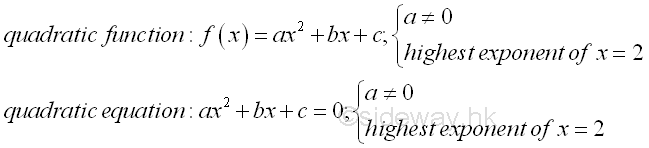The solutions of a quadratic equation is also called roots of a quadratic equation. Since a quadratic equation is a second order polynomial equation, a quadratic equation must has two solutions according to the fundamental theorem of algebra. In general, the solution may be both real, or both complex. Assume α and β are the roots of the quadratic equation, ax2+bx+c=0, the quadratic equation can then be written as (x-α)(x-β)=ax2-(α+β)x+αβ=0, imply (α+β)=-b/a and αβ=c/a by equating the coefficients of two polynomials.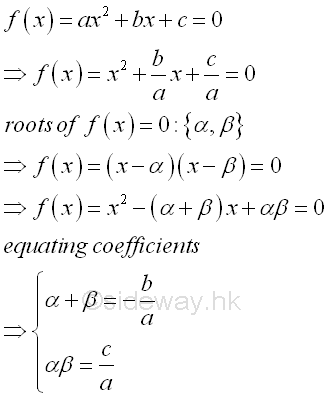Analytically, the solutions of quadratic equation can be found by the method of "completing the square". The idea of determining the roots of a quadratic equation is making use of the symmetry of the quadratic function g(x) about a vertical axis parallel to the y-axis by transforming the quadratic equation f(x)=0 into a symmetry quadratic function g(x). The obtained formula for calculating the roots of the quadratic equation is called the quadratic formula.   ImplyAssuming α≥β then the values of the α and β areAccording to the quadratic formula, the roots of a quadratic equation is charactered by the square root components of the quadratic formula. Depending on the value of expression (b2-4ac), the roots of a quadratic equation can be coincident, rational, real or complex numbers. And (b2-4ac) is therefore called the discriminant of the quadratic equation. In general, the discriminant, b2-4ac, can have four cases:

1. b2>4ac, imply discriminant, b2-4ac>0, if b2-4ac is a perfect or complete square then two distinct roots are rational.2. b2>4ac, imply discriminant, b2-4ac>0, if b2-4ac is not a perfect or complete square then two distinct roots are real or irrational.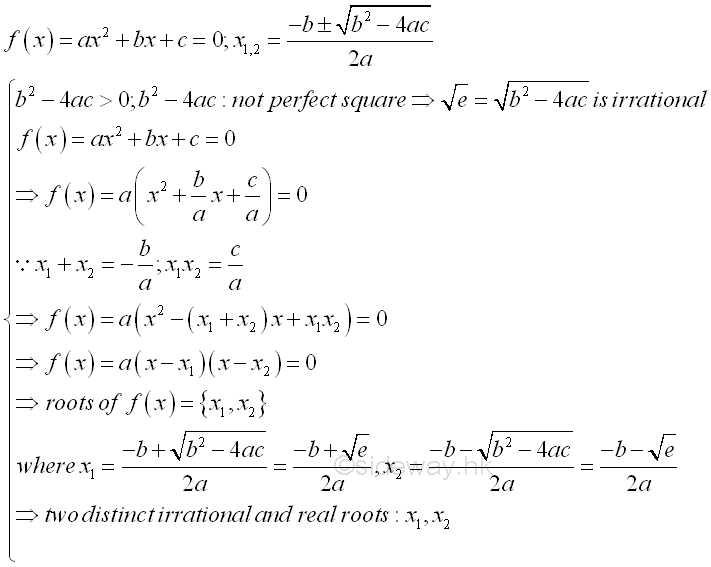3. b2=4ac, imply discriminant, b2-4ac=0, two roots are rational and coincident. That is two equal roots, one double root or one single solution.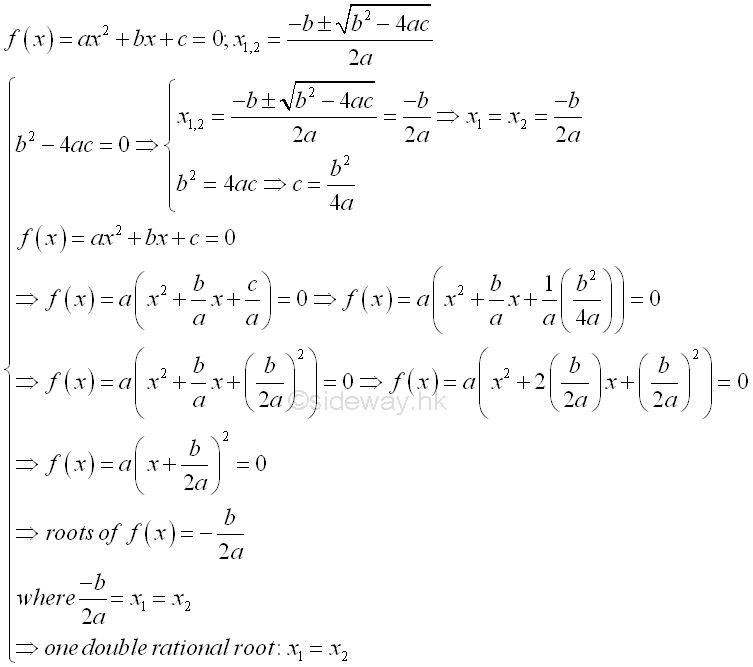4. b2<4ac, imply discriminant, b2-4ac<0, two distinct roots are complex or imaginary.## Alternate Form of Quadratic Formula

The quadratic equation is obtained by setting the quadratic function equal to zero and x is not equal to zero, the quadratic formula can be derived in an alternative way.The quadratic function f(x)=ax2+bx+c is usually called the standard form of a quadratic function. Since a quadratic equation has two roots, the standard form of a quadratic function can be expressed in terms of the two roots,  f(x)=a(x-x1)(x-x2), called factored form.Besides the quadratic function is symmetric about a line, the standard form of a quadratic function can be expressed in terms of the vertex of the function, f(x)=a(x-x0)2+y0, called vertex form.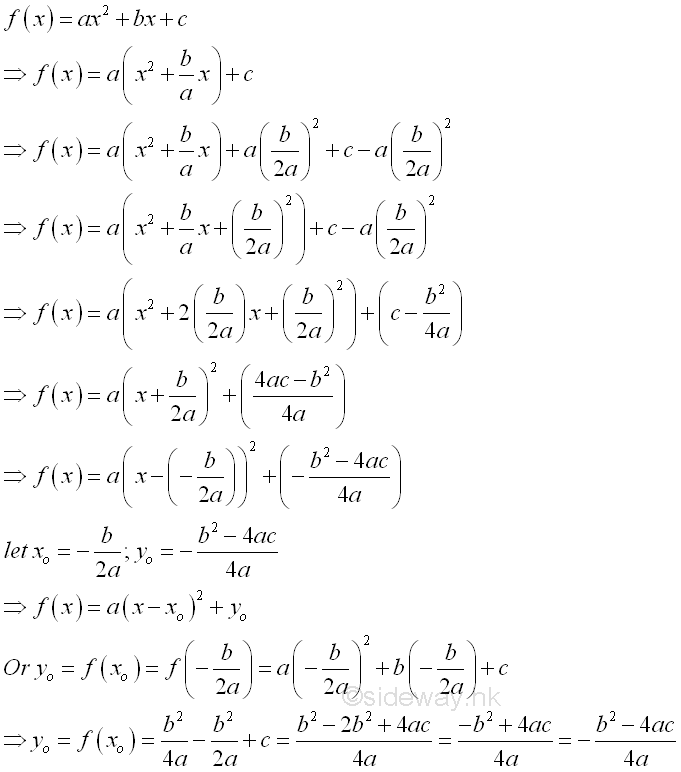Let E be the quadratic expression of the quadratic function f(x)=ax2+bx+c. If α and β are the roots of quadratic equation ax2+bx+c=0 and α≥β, then the sign of the quadratic expression can also be determined accordingly. In general, assuming the quadratic expression with two distinct real roots, when a is positive,  E is positive if x>α or x<β and E is negative if α>x>β. Or when a is negative, E is positive if α>x>β and E is negative if x>α or x<β. In other words, E has same sign as coefficient a for x>α or x<β, and E has opposite sign as coefficient a for α>x>β. This case can also be considered as the case b2-4ac>0 with two distinct real root for the corresponding quadratic equation. Imply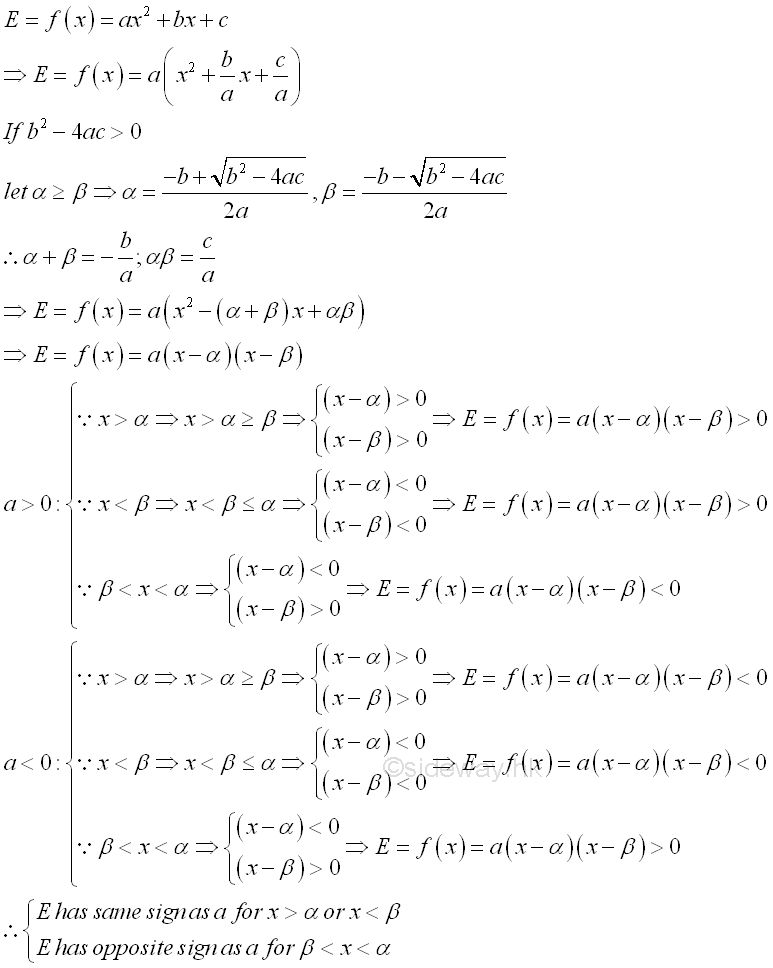In the case b2-4ac=0 with double real root  for the corresponding quadratic equation, this is a special condition of the general case where α equal to β and therefore the condition α>x>β becomes α=x=β is equal to zero. E has the same sign as the coefficient a.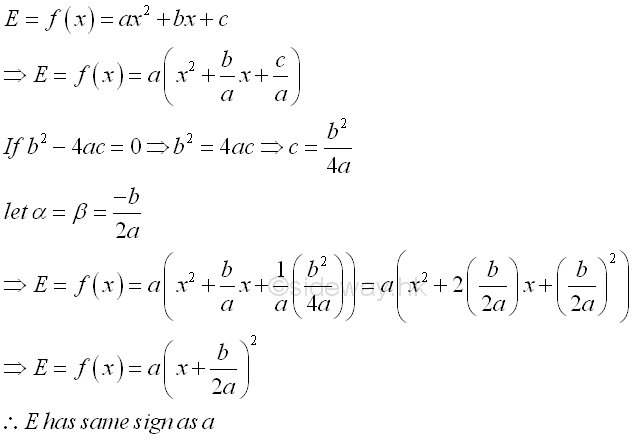In the case b2-4ac<0 with two distinct complex root  for the corresponding quadratic equation,  this is a special condition of the general case where quadratic expression, E, does not equal to zero for all real x. E has the same sign as the coefficient a..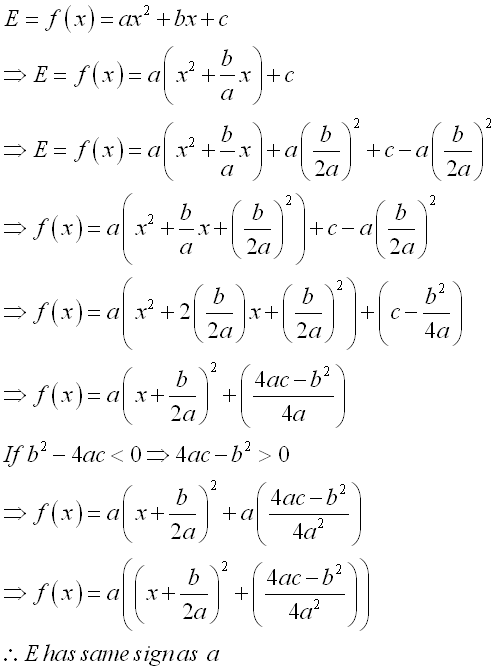ID: 130500017 Last Updated: 5/17/2013 Revision: 0 Ref:References

1. B. Joseph, 1978, University Mathematics: A Textbook for Students of Science &amp; Engineering
2. Wheatstone, C., 1854, On the Formation of Powers from Arithmetical Progressions
3. Stroud, K.A., 2001, Engineering Mathematics
4. Coolidge, J.L., 1949, The Story of The Binomial TheoremHome 5

Management

HBR 3

Information

Recreation

Culture

Chinese 1097

English 339

Reference 79Computer

Hardware 224Software

Application 213

Latex 52Manim 204

KB 1

Numeric 19

Programming

Web 289

Unicode 504

HTML 66

CSS 65

SVG 46

ASP.NET 270

OS 429

Python 72

Knowledge

Mathematics

Algebra 84

Geometry 33Calculus 67

Engineering

Mechanical

Rigid Bodies

Statics 92

Dynamics 37

Control

Natural Sciences

Electric 27